Which of these is a reducing agent ?
1. CrO3/H+
2. KMnO4
3. LiAlH4
4. O3

Concept Questions :-

Alcohols: Preparation, Physical and Chemical Properties
High Yielding Test Series + Question Bank - NEET 2020

Difficulty Level: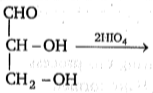Products obtained in the above reaction are :

(a) HCHO, HCO2H
(b) HCHO, 2HCO2H
(c) CO2, 2HCO2H
(d) CO2, HCHO, HCO2H

Concept Questions :-

Alcohols: Preparation, Physical and Chemical Properties
High Yielding Test Series + Question Bank - NEET 2020

Difficulty Level:

0.092 g of a compound with the molecular formula C3H8O reaction with an excess of CH3MgI gives 67.00 mL of methane at STP. The number of active hydrogen atoms present in a molecule of the compound is:
(a) one
(b) two
(c) three
(d) four

Concept Questions :-

Alcohols: Preparation, Physical and Chemical Properties
High Yielding Test Series + Question Bank - NEET 2020

Difficulty Level:

Optically active 2-octanol rapidly loses its optical activity when exposed to :

(a) dilute acid

(b) dilute base

(c) light

(d) humidity

Concept Questions :-

Alcohols: Preparation, Physical and Chemical Properties
High Yielding Test Series + Question Bank - NEET 2020

Difficulty Level: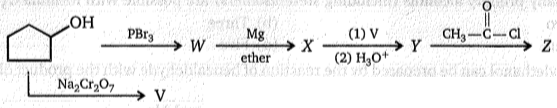Product Z of above reaction is :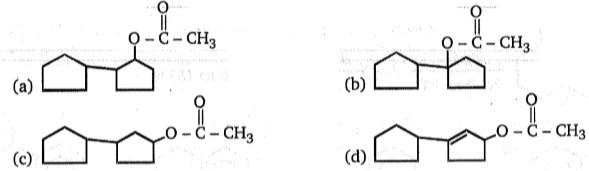Concept Questions :-

Alcohols: Preparation, Physical and Chemical Properties
High Yielding Test Series + Question Bank - NEET 2020

Difficulty Level:

Which of the following compound is hemiacetal ?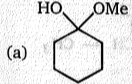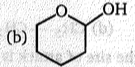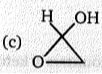(d) All of these

Concept Questions :-

Ethers: Preparation, Physical & Chemical Properties, Uses
High Yielding Test Series + Question Bank - NEET 2020

Difficulty Level:

Arrange their stabilities of given gem- diols in decreasing order.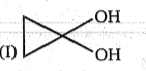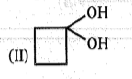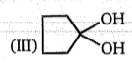(a) I > II > III

(b) III > II > I

(c) I > III > II

(d) III > I > II

Concept Questions :-

Alcohols: Preparation, Physical and Chemical Properties
High Yielding Test Series + Question Bank - NEET 2020

Difficulty Level: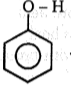$\stackrel{{\mathrm{HNO}}_{3}/{\mathrm{H}}_{2}{\mathrm{SO}}_{4}}{\to }$ (A)                  +               (B)

(more volatile)                     (less volatile)

Product (A) of the above reaction is: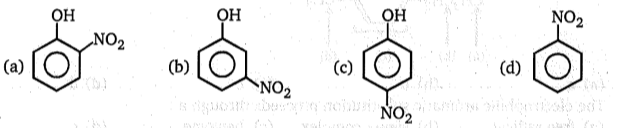Concept Questions :-

Phenols: Preparation, Properties, Acidic Nature of Phenol
High Yielding Test Series + Question Bank - NEET 2020

Difficulty Level:

What is the product obtained by heating the following allylic ether of phenol?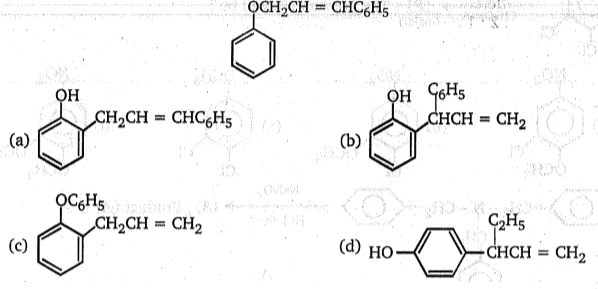Concept Questions :-

Phenols: Preparation, Properties, Acidic Nature of Phenol
High Yielding Test Series + Question Bank - NEET 2020

Difficulty Level: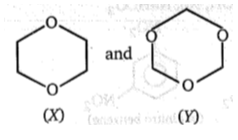; Compounds (X) and (Y) can be differentiated by:
(a) ${\mathrm{H}}_{3}{\mathrm{O}}^{\oplus }$, NaOI
(b) ${\mathrm{H}}_{3}{\mathrm{O}}^{\oplus }$, then Fehling test
(c) ${\mathrm{H}}_{3}{\mathrm{O}}^{\oplus }$, then Na
(d) Both (b) and (c)

Concept Questions :-

Ethers: Preparation, Physical & Chemical Properties, Uses